# Gravitational field strength

July 11, 2023After studying this section you should be able to:

• understand the concept of a field
• recall and use the relationship that describes the gravitational force between two masses
• describe the Earth’s gravitational field and explain how the field strength varies with distance from the centre of the Earth

The following sections can be found below

Fields

A field is a region of space where forces are exerted on objects with certain properties. In this and subsequent sections three types of field are considered:

• gravitational fields affect anything that has mass
• electric fields affect anything that has charge
• magnetic fields affect permanent magnets and electric currents.

These three types of field have many similar properties and some important differences. There are key definitions and concepts that are common to all three types of field.

Radiant energy such as light has mass and so is affected by gravitational fields.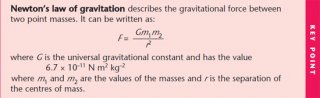Gravitational fields

Newton realised that all objects with mass attract each other. This seems surprising, since any two objects placed close together on a desktop do not immediately move together. The attractive force between them is tiny, and very much smaller than the frictional forces that oppose their motion.

Gravitational attractive forces between two objects only affect their motion when at least one of the objects is very massive. This explains why we are aware of the force that attracts us and other objects towards the Earth – the Earth is very massive. The mass of the Earth is

The diagram represents the Earth’s gravitational field. The lines show the direction of the force that acts on a mass that is within the field.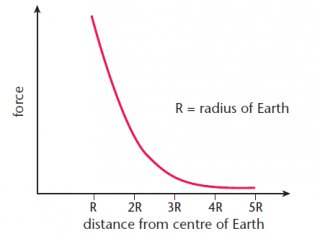This diagram shows that:

• gravitational forces are always attractive – the Earth cannot repel any objects
• the Earth’s gravitational pull acts towards the centre of the Earth
• the Earth’s gravitational field is radial; the field lines become less concentrated with increasing distance from the Earth.

The force exerted on an object in a gravitational field depends on its position. The less concentrated the field lines, the smaller the force. If the gravitational field strength at any point is known, then the size of the force can be calculated.

Gravitational field strength is a vector quantity: its direction is towards the object that causes the field.

Always use the given value for g. Candidates often lose marks for using 10ms–2 when the formula sheet gives g = 9.81ms–2

Universal gravitation

In studying gravitation, Newton concluded that the gravitational attractive force that exists between any two masses:

• is proportional to each of the masses
• is inversely proportional to the square of their distances apart.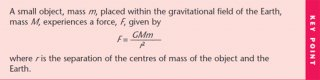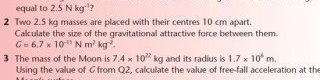Source: revisionworld.com
##### INTERESTING VIDEO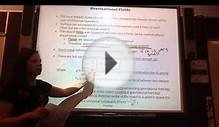Gravitational Field Strength part 1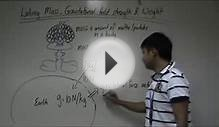O-Phy-4 Mass, Gravitational Field Strength and Weight ...6 Changes in Gravitational Field Strength